# Lecture 7: MCMC¶

AM207: Pavlos Protopapas, Harvard University

Feb 18 2014

# A little history¶

In 1946, Stan Ulam while recovering from an illness was playing solitaire and he was trying to find the 'perfect' game by computing the chances that a particular solitaire laid out with 52 cards would come out successfully. He attempted combinatorial calculations but eventually he decided to lay out several solitaires at random and observe the number of successful plays. This idea of selecting a statistical sample to approximate a hard combinatorial problem by a much simpler problem is at the heart of modern Monte Carlo simulation.

Ulam realized that computers could be used to answer questions of many physics problems (in particular neutron diffusion). With the help of John Von Neumann in the next few years developed many Monte Carlo algorithms, including importance sampling and rejection sampling. [Enrico Fermi in the 1930s also used Monte Carlo in the calculation of neutron diffusion, and later designed the FERMIAC, a Monte Carlo mechanical device that performed calculations]. In the 1940s Nick Metropolis was fascinated with Monte Carlo methods and designed new controls for the state-of-the-art computer (ENIAC) with Klari Von Neumann, John's wife. He also designed an improved computer, which he named the MANIAC in the hope that computer scientists would stop using acronyms. This computing machines became popular amongst many mathematicians and physicists (Fermi, Von Neumann, Ulam, Teller, Richtmyer, Bethe, Feynman, and Gamow) that would go ask him to help them with their problems. In 1949, he published the first public document on Monte Carlo simulation with Stan Ulam (Metropolis and Ulam, 1949). This paper introduced, among other ideas, Monte Carlo particle methods which we will see in later lectures. He proposed the Metropolis algorithm with the Tellers and the Rosenbluths (Metropolis et al., 1953).

Metropolis algorithm is among the top ten most used algorithms in science and engineering in the last twenty years. Metropolis algorithm is one of the algorithms in a large class of stochastic algorithms known as Markov chain Monte Carlo (MCMC). These algorithms are often used in statistics, econometrics, physics and computing science, etc and have proven to make significant contribution to many scientific fields. Especially in high-dimensional problems, such as computing the volume of a convex body in d-dimensions, for which MCMC simulation is the only known general approach for providing a solution within a reasonable time (polynomial in d).

The most significant paper after this was the generalization of the Metropolis algorithm by Hastings in 1970. Hastings and his student Peskun showed that Metropolis and the more general Metropolis-Hastings algorithms are particular instances of a large family of algorithms. They studied the optimality of these algorithms and introduced the formulation of the Metropolis-Hastings algorithm that we look in these lectures.

There are a few MCMC publications in the statistics literature but it is not until 1990 that MCMC made the first impact in statistics (Gelfand & Smith, 1990).

In :
%matplotlib inline
import numpy as np
import matplotlib.pylab as plt
import pandas as pd

pd.set_option('display.width', 500)
pd.set_option('display.max_columns', 100)
import seaborn as sns


# Introduction¶

We are all used to using functions. In the last lectures instead of just using the posterior pdf, which is a function that we can use all the nice tools you know from calculus you learned in kindergarten, I, all of sudden started returned 'random' numbers at you. Why we are returned thousands of samples from the posterior as a solution, which at first is confusing.

We also learned how to generate samples from posteriors (or priors) with the rejection method and that is what we used so far (lectures and homework). We also know that importance sampling is better but to use that we need to know the normalization. The wish is to do importance sampling without having to know the normalization, and that is what MCMC is all about.

Problems that are intractable using analytic approaches often become possible to solve using some form of MCMC, even with high-dimensional problems. The development of MCMC is arguably the biggest advance in the computational approach to statistics. While MCMC is very much an active research area, there are now some standardized techniques that are widely used. We will discuss many forms of MCMC: Metropolis-Hastings, Gibbs sampling, slice sampling, Hamiltonian MCMC and few others. Before we go into these techniques though, we ﬁrst need to understand the two main ideas underlying MCMC: Monte Carlo integration (we have done this), and Markov chains

### Motivation¶

MCMC techniques are applied to solve integration and optimization problems in large dimensional spaces. These two types of problem play a fundamental role in machine learning, physics, statistics, econometrics and decision analysis. For example

• Bayesian inference and learning
• Optimization
• Statistical mechanics

### MCMC Algorithm¶

MCMC is a strategy for generating samples $x^{(i)}$ using a Markov chain mechanism. This mechanism is constructed so that the chain spends more time in the most important regions. In particular, it is constructed so that the samples $x^{(i)}$ mimic samples drawn from the target distribution $p(x)$. We should emphasize that we use MCMC when we cannot draw samples from $p(x)$ directly, but can evaluate $p(x)$ up to a normalization constant.

# Markov Chains¶

For simplicity we should introduce Markov chains on finite state space, where $x^{(i)}$ can only take discrete values $x^{(i)} =\left\{x_1^{i},x_2^{i}, \ldots, x_s^i \right\}$. This stochastic process is called Markov chain if we transition from one state to another state using a simple sequential procedure. We start a Markov chain at some state $x^{1}$, and use a transition function $T(x^{(i)} | x^{(i-1)})$, to determine the next state, $x^{(2)}$ conditional on the last state. We then keep iterating to create a sequence of states:

$$x^{(1)} \rightarrow x^{(2)} \rightarrow \ldots \rightarrow x^{(i)} \rightarrow \ldots$$

Each such a sequence of states is called a Markov chain or simply chain. The procedure for generating a sequence of T states from a Markov chain is the following:

• Set $i=1$
• Generate a initial value $u$, and set $x^{(1)} = u$
• Repeat

1. $i=i+1$
2. Sample a new value u from the transition function $T(x^{i}| x^{(i-1)})$
3. Set $x^{(i)} = u$

4. Until $i=T$

Importantly, in this iterative procedure, the next state of the chain at $i + 1$ is based only on the previous state at $i$. Therefore, each Markov chain wanders around the state space and the transition to a new state is only dependent on the last state. It is this local dependency what makes this procedure Markov or memoryless. This is an important property when using Markov chains for MCMC. Formally

$$p(x^{(i)} | x^{(i-1)}\ldots x^{(1)}) = T(x^{i}| x^{(i-1)})$$

The chain is called homogenous if $T(x^{i}|x^{(i-1)})$ remains unchanged for all $i$. In other words the evolution of the chain depends only on the current state of the chain and a invariant transition matrix.

As an example consider a life insurance company that wants to find out how much money to charge its clients. The company wants to estimate how long the clients will live. It proposes the following model summarizing the state of health of an individual on a monthly basis: healthy, sick and dead with transition graph shown in figure belowThe transition matrix for this example is

$$\left[ \begin{array}{ccc} 0.69 & 0.3 & 0.01 \\ 0.8 & 0.1 & 0.1 \\ 0 & 0 & 1 \end{array} \right]$$
If the probability vector for the initial state is $\mu(x^{(1)}) = (0.5, 0.2, 0.3)$ it follows that $\mu(x^{(1)})T = (0.5050, 0.1700, 0.3250)$ and, after several iterations (multiplications by T ), the product will converge to $p(x) =(0.0178,0.0062,0.9760)$ [all dead]. No matter what initial distribution we use, the chain will stabilize at $p(x) = (0.0, 0.0, 1.0)$.

When initializing each Markov chain, the chain will wander in state space around the starting state. Therefore, if we start a number of chains, each with different initial conditions, the chains will initially be in a state close to the starting state. This period is called the burnin. An important property of Markov chains is that the starting state of the chain no longer affects the state of the chain after a sufficiently long sequence of transitions (assuming that certain conditions about the Markov chain are met). At this point, the chain is said to reach its steady state and the states reflect samples from its stationary distribution. This property that Markov chains converge to a stationary distribution regardless of where we started (if certain regularity conditions of the transition function are met), is quite important. When applied to MCMC, it allow us to draw samples from a distribution using a sequential procedure but where the starting state of the sequence does not affect the estimation process.

In :
# Simple example for MARKOV CHAIN
# transition matrix
T = np.array([[0.69, 0.3, 0.01],[ 0.8, 0.1, 0.1] ,[ 0,0.1 ,0.9]])

# initial state USE RANDOM NUMBERS
p = np.random.uniform(low=0,high=1, size=3)
p = p/np.sum(p)

q=np.zeros((100,3))
for i in np.arange(0,100):
q[i, :] = np.dot(p,np.linalg.matrix_power(T,i))

plt.plot(q)
plt.xlabel('i')
plt.legend( ('H', 'S', 'D'))

Out:
<matplotlib.legend.Legend at 0x10969c350>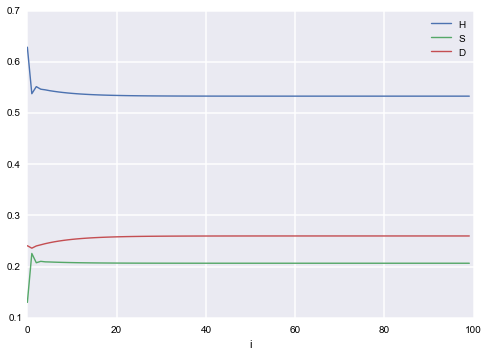This stability result plays a fundamental role in MCMC simulation. For any starting point, the chain will convergence to the invariant distribution $p(x)$, as long as $T$ is a stochastic transition matrix that obeys the following properties:

• Irreducibility. For any state of the Markov chain, there is a positive probability of visiting all other states.
• Aperiodicity. The chain should not get trapped in cycles.
• Invariance. A condition to ensure that a particular $p(x)$ is the desired invariant distribution is the following reversibility (detailed balance) condition
$$p(x^{(i)}) \, T(x^{(i-1)} | x^{(i)}) = p( x^{(i-1)}) \, T(x^{(i)} | x^{(i-1)})$$

and if we sum both sides over $x^{(i-1)}$, it produces

\begin{equation} p(x^{(i)}) = \sum_{x^{(i-1)}} p( x^{(i-1)}) \, T(x^{(i)} | x^{(i-1)}) \end{equation}

because $\sum_{x^{(i-1)}} T(x^{(i-1)} | x^{(i)}) = 1$

### Hint: Transition matrix properties, the good and the bad¶

If a matrix has at least one eigenvalue of -1 then the solution as t→∞, oscillates. To construct a matrix that has certain eigenvalues we can use M=R∗D∗R−1 where R is an invertible matrix and D is a diagonal square matrix with its diagonal values equal to the eignevalues.

### Example¶

The concepts of irreducibility, aperiodicity and invariance can be better realized when we search for information on the web.

Google computes a value called PageRank for each entry in its search engine. PageRank essentially measures how important or valuable a particular website/webpage is. PageRank is part of what determines which web page is displayed in which rank when you hit the Search button. For some page ${\cal P}$, PageRank uses the number of pages that link to ${\cal P}$, as well as the number of pages that ${\cal P}$ links to compute the metric. For some page ${\cal P}$, the PageRank is defined as

$$PR( {\cal P}) = \sum_{{\cal V}} \frac{PR({\cal V})}{L({\cal V})}$$

PageRank assumes that the user randomly surfs from page ${\cal V}$ to $\cal P$ and it depends only on the previous page visited and no other page. This hints at a Markov chain. Google is able to estimate the PageRank values using MCMC. After a certain number of iterations, the Markov chain converges to a stationary distribution of PR values that approximate the probability that a user would randomly surf to some page $\cal P$.

## MCMC¶

We have discussed Monte Carlo sampling and Markov chains. Monte Carlo sampling is design to estimate various characteristics of a distribution such as the mean, variance, kurtosis, or any other statistic. Markov chains involve a stochastic sequential process where we can sample states from some stationary distribution. The goal of MCMC is to design a Markov chain such that the stationary distribution of the chain is exactly the distribution that we are interesting in sampling from. This is called the target distribution. In other words, the states sampled from the Markov chain should follow the same statistics of samples drawn from the target distribution. The idea is to use some clever methods for setting up the proposal distribution such that no matter how we initialize each chain, we will convergence to the target distribution.

## The Metropolis-Hastings algorithm¶

$$\newcommand{\var}{{\rm var}} \newcommand{\cov}{{\rm cov}} \newcommand{\corr}{{\rm corr}} \newcommand{\xss}{x^{(i+1)}} \newcommand{\xs}{x^{\star}} \newcommand{\xt}{x^{(i)}} \newcommand{\xa}{x_a} \newcommand{\xb}{x_b}$$

The Metropolis-Hastings (MH) algorithm is the most popular of the MCMC methods (Metropolis et al 1953, Hastings 1970). As a matter of fact we will see that most MCMC algorithms can be interpreted as special cases of this algorithm.

Suppose our goal is to sample from the target density $p(x)$, with $-\infty< x < \infty$ . The Metropolis-Hastings sampler creates a Markov chain that produces a sequence of values: $$x^{(1)} \rightarrow x^{(2)} \rightarrow \ldots \rightarrow x^{(t)} \ldots$$ where $x^{(t)}$ represents the state of a Markov chain at iteration $t$. The samples from the chain, after burnin, reflect samples from the target distribution $p(x)$.

The MH algorithm, initializes the first state, $x^{(1)}$ to some initial value, and then uses a proposal distribution $q(\xs|\xt)$ to sample a candidate value $\xs$ given the current value of $\xt$ according to $q(\xs|\xt)$. The Markov chain then moves towards $\xs$ with the acceptance probability

$$A(\xt,\xs) = \min\left[1, \frac{p(\xs) \, q(\xt|\xs)}{p(\xt) q(\xs|\xt) } \right]$$

otherwise it remains at $\xt$.

• Initialize $x^{(1)}$
• For i=2 to N

• Sample $u\sim U(0,1)$
• Sample $\xs \sim q(\xs|x^{(i)})$
• if $u<A(x^{(i)},\xs) = \min\left[1, \frac{p(\xs) \, q(x^{(i)}|\xs)}{p(x^{(i)}) q(\xs| \xt ) } \right]$

$x^{(i+1)}=\xs$

• else
$x^{(i+1)}=x^{(i)}$

Note that if the transition probability is symmetric $q(\xs|x^{(i)}) = q(x^{(i)}|\xs)$ then the acceptance probability becomes

$$A(x,\xs) = \min\left[1, \frac{p(\xs)}{p(x) } \right]$$

which was the original algorithm proposed by Metropolis, thus the Metropolis algorithm.

A major advantage of the MH sampler is that the equation above involves only a ratio of densities. Therefore, any terms independent of $x$ in the functional form of $p(x)$ will drop out. Therefore, we do not need to know the normalizing constant of the density or probability. The fact that this procedure allows us to sample from unnormalized distributions is one of its major attractions. Sampling from unnormalized distributions frequently happens in Bayesian models, where calculating the normalization constant is difficult or impractical.

The MH algorithm is very simple, but it requires careful design of the proposal distribution $q(\xs| x)$. In subsequent lectures, we will see that many MCMC algorithms arise by considering specific choices of this distribution.
A way to design these sampling mechanism is to ensure that detailed balance is satisfied. However, it is also important to design samplers that converge quickly or the burnin time is short. Indeed, most of the effort will be dedicated to increasing the convergence speed.

## Examples¶

### Example1¶

In this example we sample from $p(x) =6x(1-x)$ using the Metropolis algorithm with proposal distribution

$$\xs \sim N(x, 0.6)$$
In :
## FUNCTIONS
# target distribution p(x)
p = lambda x: 6*x*(1-x)

# number of samples
n = 100000

sig =0.10

#intitialize the sampling. Start somewhere from 0..1
x0 = np.random.uniform()

x_prev = x0

x=[]
k=1
i=0
while i<n:

x_star = np.random.normal(x_prev, sig)
while  (x_star <0) | (x_star > 1):  # MAKE SURE WE STAY WITHIN BOUNDS
x_star = np.random.normal(x_prev, sig)

P_star = 6*x_star*(1-x_star) #p(x_star);
P_prev = 6*x_prev*(1-x_prev) #p(x_prev);
U =  np.random.uniform()

A =  P_star/P_prev
if U < A:
x.append(x_star)
i = i + 1
x_prev = x_star
else :
x.append(x_prev)
x_prev = x[i]
i = i + 1

k=k+1

e,q,h=plt.hist(x,10, alpha=0.4, label=u'MCMC distribution')

xx= np.linspace(0,1,100)
plt.plot(xx, 0.67*np.max(e)*p(xx), 'r', label=u'True dsitribution')
plt.legend()

Out:
<matplotlib.legend.Legend at 0x109039a10>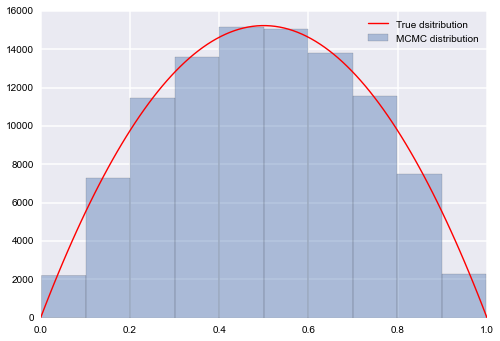In :



### The normal distribution¶

In this example we sample from normal distribution (assuming we do not know how to do this) using the Metropolis algorithm with a proposal distribution

$$\xs \sim U( x-\delta, x+\delta)$$

where $\delta=0.5$ is the random walk step.

In :
plt.figure(figsize=[12,6])

from scipy.stats import norm
# the target distribution
p = lambda x: norm.pdf(x)

delta = .5
# proposal distribution rule (random -delta .. delta)
q = lambda x: x + np.random.uniform()*2*delta - delta;

# number of samples
n = 10000

# start somewhere from 0..1
x0 = np.random.uniform()
x=np.zeros(n)

x_prev = x0

k=1
i=1
while i<n:

x_star = q(x_prev)

P_star = p(x_star)
P_prev = p(x_prev)
U = np.random.uniform()

A = np.min((1, P_star/P_prev))

if U <= A:
x[i] = x_star
i = i + 1
x_prev = x_star
else:
x[i] = x_prev
x_prev = x[i]
i = i + 1
k=k+1

# =======
# PLOTTING
plt.subplot(1,2,1)
fig = plt.hist(x, 20, alpha=0.3)
fig = plt.hist(x[300:], 20, alpha=0.3)

plt.subplot(1,2,2)
plt.plot(x)

Out:
[<matplotlib.lines.Line2D at 0x1090cf6d0>]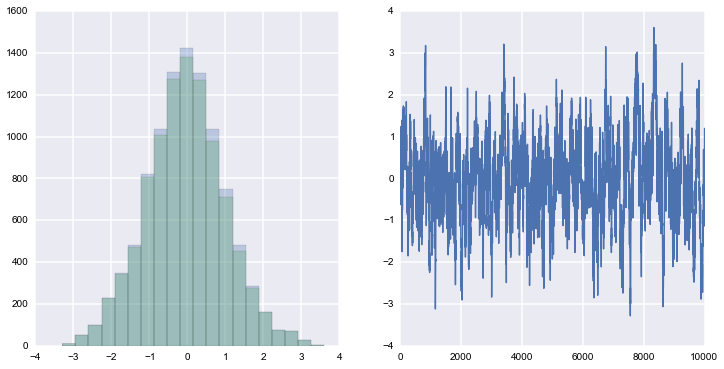The fact that asymmetric proposal distributions can be used allows the Metropolis-Hastings procedure to sample from target distributions that are defined on a limited range (other than the uniform for which Metropolis sampler can be used). With bounded variables, care should be taken in constructing a suitable proposal distribution. Generally, a good rule is to use a proposal distribution that has positive density on the same support as the target distribution. For example, if the target distribution has support over $0 \le x < \infty$, the proposal distribution should have the same support.

## Why does MH work?¶

Starting from the ergodic theorem: If $X=(x^{(1)}, x^{(2)}, \ldots, \xt)$ is an irreducible, aperiodic, recurrent Markov chain, then there is a unique probability $\pi$ such as $i\rightarrow \infty$

$$p(\xt \in A) \rightarrow \pi(A)$$

for any $A$ where $\pi(x)$ is the stationary distribution of the Markov chain. It is not hard to generate aperiodic, irreducible and recurrent Markov chains. To prove that the Markov chain is recurrent or having a stationary distribution follows from above.

What we need to prove is that the stationary distribution is the target distribution.

For simplicity assume $X$ is discrete.
Say $\xt$ is sampled from the target distribution $p(x)$ and if $\xss$ is generated from $\xt$ using MH, then if we can prove that $p(\xss)=p(x)$ we know that the stationary distribution is the target distribution.

Let $\xa$ and $\xb$ be any two values of $X$ such that: $p(x_a) \, q(x_b \vert x_a) >= p(x_b) q(x_a \vert x_b)$, then under the MH algorithm the probability that

$$\xt = \xa \hspace{0.3cm} {\rm and} \,\,\, \xss = \xb$$

is equal to the probability of

• sampling $\xt=\xa$ from $p(x)$
• proposing $\xs=\xb$ from $q(\xs | \xt)$
• accepting $\xss=\xb$

The probability of these three things to happen is the product of probabilities

$$p(\xt = \xa, \xss = \xb)=p(\xa) \times q(\xb|\xa) \times \frac{p(\xb) \, q(\xa|\xb)}{p(\xa) \,q(\xb|\xa)} =p(\xb) \, q(\xa|\xb)$$

On the other hand the probability that $\xt =\xb$ and $\xss = \xa$ is the probability that $\xb$ is sampled from $p$, $\xa$ is proposed from $q(\xs|\xt))$ and $\xa$ is accepted as $\xss$. But remember we assumed

$$p(\xa) \, q(\xa|\xb) \ge p(\xb) \, q(\xb|\xa)$$

which means the acceptance probability is 1 and therefore

$$p(\xt = \xb, \xss = \xa) = p(\xb) \, q(\xa|\xb)$$

$$\Rightarrow p(\xt = \xb, \xss = \xa) =p(\xt = \xa, \xss = \xb)$$ Now lets consider the marginalized probability

$$p(\xss =x) = \sum_{\xa} p(\xss = x, \xt = \xa) =\sum_{\xa} p(\xss = \xa, \xt = x) = p(\xt = x)$$

Which means that $p(\xss=x) = p(x)$ if $p(\xt=x) = p(x)$.

Alternative proof: Consider a large number of walkers' starting from different initial points and moving independently of each other. Let $N_n(X)$ be the number of walkers at $X$ after $n$ steps, then the net number of walkers moving from point $X$ to a new point $Y$ is $$\Delta N_n(X) = N_n(X) P(X\rightarrow Y) - N_n(Y) P(Y \rightarrow X)$$ $$= N_n(Y)P(X\rightarrow Y) \left[ \frac{N_n(X)}{N_n(Y)} -\frac{P(Y\rightarrow X) }{P(X\rightarrow Y)} \right]$$ where $P(X\rightarrow Y)$ is the probability that a walker at $X$ will move to $Y$. When

$$\frac{N_c(X)}{N_c(Y)} =\frac{N_n(X)}{N_n(Y)} = \frac{P(Y\rightarrow X) }{P(X\rightarrow Y)}$$

then the number of walkers at point $X$ remains constant and therefore the system is at steady state or the system is at the invariant distribution.

The probability that a walker at $X$ will move to $Y$ is equal to the probability of proposing the point $Y$ being at point $X$, times the probability of accepting that proposed new position

$$P(X\rightarrow Y) = q(Y|X) \, A(X,Y)$$

Lets assume the transition probability is symmetric, therefore

$$\frac{N_c(X)}{N_c(Y)} = \frac{A(Y,X)}{A(X,Y)}$$

If $p(X) < p(Y)$ then we accept $Y$ with probability one, $A(Y,X)=1$, and $A(X,Y) = p(Y)/p(X)$. On the other hand if $p(X) > p(Y)$ then we accept $X$ with probability one, $A(X,Y)=1$ and $A(Y,X) = p(X)/p(Y)$. In conclusion

$$\frac{N_c(X)}{N_c(Y)} = \frac{p(X)}{p(Y)}$$

in both cases, which means the probability density of any point $X$ is the same as the target pdf.

## Metropolis-Hastings for Multivariate Distributions¶

Up to this point, we discussed univariate distributions. It is easy though to generalize the MH sampler to multivariate distributions. There are two different ways to extend the procedure to sample random variables in multidimensional spaces.

## Blockwise updating¶

Blockwise updating, uses a proposal distribution that has the same dimensionality as the target distribution. If the probability distribution involves $N$ variables, we design a N-dimensional proposal distribution, and we either accept or reject the proposal (involving values for all N variables) as a block. In the following, we will use the vector notation ${\bf x} = (x_1, x_2,\ldots, x_N)$ to represent a random variable involving N components, and $\xt$ to represents the i-th state in our sampler. We then simply replace the scalar $x$ with ${\bf x}$ in the MH sampler. In summary

• Set i=1
• Generate ${\bf u} = (u_1, u_2, \ldots, u_N)$ and set ${\bf x^{(1)}} = {\bf u}$
• Repeat

1. Propose ${\bf \xs}$ from $q({\bf \xss}|{\bf \xt)}$
2. Evaluate acceptance probability $A = \min \left( 1, \frac{p({\bf \xs})}{p({\bf \xt})} \frac{q({\bf \xt}| {\bf \xs})}{q({\bf \xs}| {\bf \xt})} \right)$
3. Generate $u \sim U(0,1)$
4. if $u\le A$ accept then ${\bf \xss} = {\bf \xs}$ else ${\bf \xss} = {\bf \xt}$

## Componentwise updating¶

A problem with the blockwise updating approach is that it might be difficult to find suitable high-dimensional proposal distributions. A related problem is that blockwise updating can be associated with high rejection rates. Instead of accepting or rejecting a proposal for $x$ involving all its components simultaneously, it might be computationally simpler to make proposals for individual components of $x$, one at a time. This leads to a componentwise updating approach.

Suppose we have a bivariate distribution, ${\bf x} = (x_1, x_2)$. First we initialize the chain with some values $x_1^{(1)}$ and $x_2^{(1)}$. We then update the chain one component at a time. We propose a $\xs_1$ and evaluate the acceptance probability depending on $\xt_i$ and then evaluate the ratio of acceptance probabilities of $(\xs_1, \xt_2)$ and $(\xt_1, \xt_2)$. In the next step, we make a proposal for $\xs_2$ depending on the $\xt_2$ and compare the ratio of $(\xss_1, \xs_2)$ and $(\xss_1, \xt_2)$. In this step, we hold the first component constant. Therefore, the second step is conditional on what happens from the first step. In summary:

• Set i=1
• Generate ${\bf u} = (u_1, u_2, \ldots, u_N)$ and set ${\bf x^{(1)}} = {\bf u}$
• Repeat

1. Propose $\xs_1$ from $q(\xss_1|\xt_1)$
2. Evaluate acceptance probability $A = \min \left( 1, \frac{p(\xs_1, \xt_2)}{p(\xt_1, \xt_2)} \frac{ q(\xt_1| \xs_1)}{q(\xs_1| \xt_1)} \right)$
3. Generate $u \sim U(0,1)$
4. if $u\le A$ accept then $\xss_1 = \xs_1$ else $\xss_1 = \xt_1$
5. Propose $\xs_2$ from $q(\xss_2|\xt_2)$
6. Evaluate acceptance probability $A = \min \left( 1, \frac{p(\xss_1, \xs_2)}{p(\xss_1, \xt_2)} \frac{q(\xt_2| \xs_2)}{q(\xs_2| \xt_2)} \right)$
7. Generate $u \sim U(0,1)$
8. if $u\le A$ accept then $\xss_2 = \xs_2$ else $\xss_2 = \xt_2$

### Example¶

Suppose we want to sample from the bivariate normal distribution

In :
xmin =-2; xmax = 2
ymin =-2; ymax = 2

sig_x = 0.1
sig_y = 0.1

n = 90000
# FUNCTIONS
p= lambda x,y:  np.exp(-x**2/2.0) * np.exp(-y**2/2.)

## NOW LETS BUILD OUR MH
# start from a random position
np.random.seed(seed=10)
x0=np.random.normal()
y0=np.random.normal()
x_prev =x0
y_prev =y0

x = np.zeros(n)
y = np.zeros(n)
i=0
while i<n:

# take a random step ~N(0,1) - proposal first in X
x_star = np.random.normal(x_prev, sig_x)

P_star = p(x_star,y_prev)
P_prev = p(x_prev, y_prev)
A =  P_star/P_prev   # Acceptance probability. Since q is symmetric I do Metropolis
U = np.random.uniform();

if (U < A):
x[i] = x_star
i = i + 1
x_prev = x_star
else:
x[i] = x_prev
x_prev = x[i]
i = i + 1

i=i-1
# now lets do the y step
y_star =  np.random.normal(y_prev, sig_y)

P_star = p(x_prev,y_star)
P_prev = p(x_prev, y_prev)
A =  P_star/P_prev

U = np.random.uniform()

if U < A:
y[i] = y_star
i = i + 1
y_prev = y_star
else:
y[i] = y_prev
y_prev = y[i];
i = i + 1

x_mean= np.mean(x)
y_mean= np.mean(y)
np.std(x)
np.std(y)

Out:
1.0046441376573734
In :
# PLOTTING
plt.figure(figsize=[12,12])
counts,xd,yd,fig=plt.hist2d(x,y,31, cmap="Purples");
#or try plt.hexbin(x,y, cmap="Purples")

xt,yt = np.meshgrid(np.linspace(-3,3, 100),np.linspace(-3,3,100))

plt.contour(xt,yt,  np.exp(-xt**2/2) * np.exp(-yt**2/2))

plt.scatter(x,y, 0.1, alpha=0.2, cmap="BuGn")

Out:
<matplotlib.collections.PathCollection at 0x10afc1950>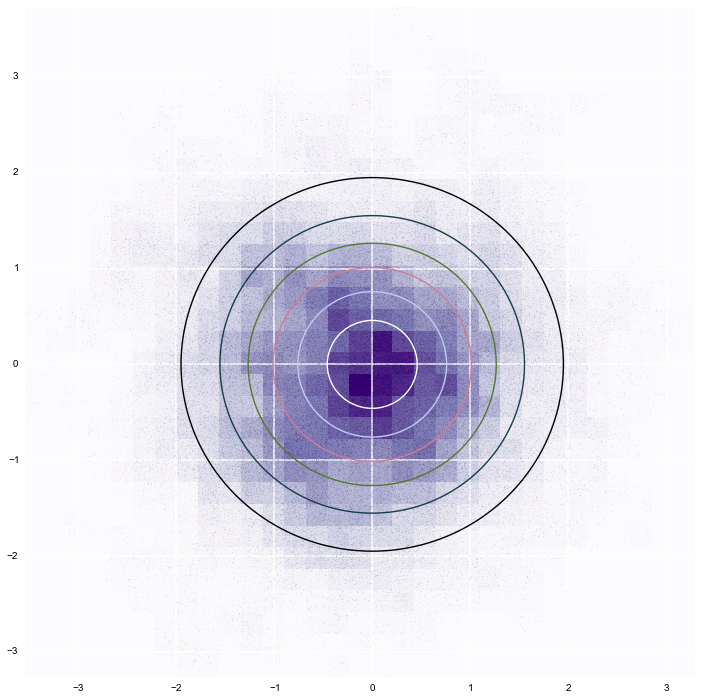In :
plt.figure(figsize=[12,12])
H, xedges, yedges = np.histogram2d(x, y, bins=(30,30))

extent = [yedges, yedges[-1], xedges[-1], xedges]

plt.imshow(H, extent=extent, interpolation='nearest', cmap="BuGn")
xt,yt = np.meshgrid(np.linspace(-3,3, 100),np.linspace(-3,3,100))
#plt.contour(xd[1:],yd[1:],counts, alpha=0.3)

plt.contour(xt,yt,  np.exp(-xt**2/2) * np.exp(-yt**2/2), cmap="BuGn")

Out:
<matplotlib.contour.QuadContourSet instance at 0x10ada5cf8>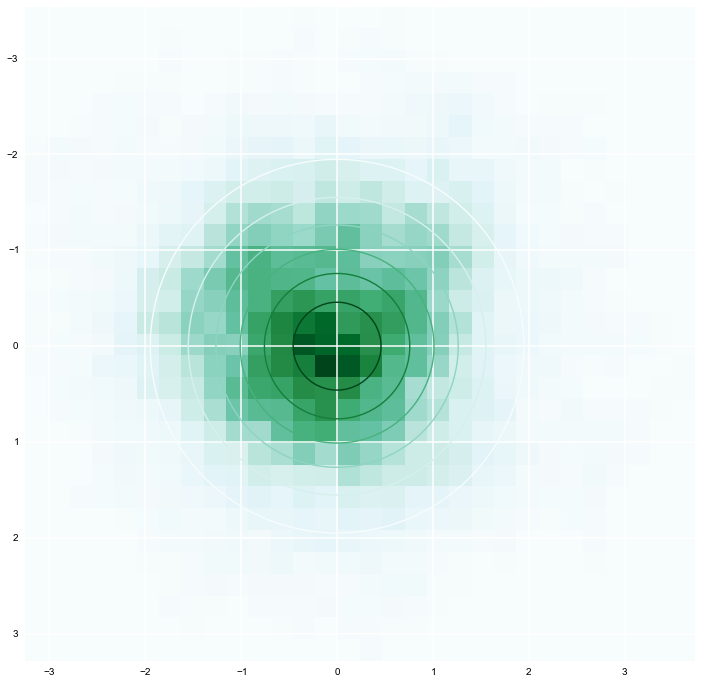In [ ]:

`